## Laplace Transform Partial Fraction:

Most transform methods depend on the partial fraction of a given transform function. Given any solution of the form N(s) = P(s)/Q(s), the inverse Laplace transform can be determined by expanding it into partial fractions. The Laplace Transform Partial Fraction depend on the type of factor. It is to be assumed that P(s) and Q(s) have real coefficients and contain no common factors. The degree of P(s) is lower than that of Q(s).

Case 1:

When roots are real and simple

In this case

N(s) = P(s)/Q(s)

where

Q(s) = (s – a)(s – b)(s – c)

Expanding N(s) into partial fractions, we get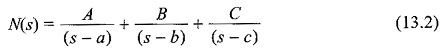To obtain the constant A, multiplying Eq. 13.2 with (s — a) and putting s = a, we get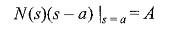Similarly, we can get the other constants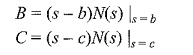Case 2:

When roots are real and multiple

In this case

N(s) = P(s)/ Q(s)

where

Q(s) = (s – a)n Q1(s)

The partial fraction expansion of N(s) is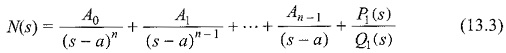where P1(s)/Q1(s) = R(s) represents the remainder twins of expansion. To obtain the constant A0,A1,…, An-1, let us multiply both sides of Eq. 13.3 by (s – a)n

Thuswhere R(s) indicates the remainder terms.

Putting s = a, we get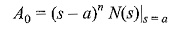Differentiating  Eq. 13.4 with respect to s, and putting s = a, we get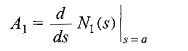Similarly,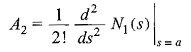In general,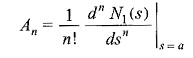Case 3:

When roots are complex

Consider a function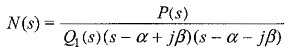The partial fraction expansion of N(s) is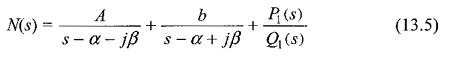where P1(s)/Q1(s) is the remainder term.

Multiplying Eq. 13.5 by (s – α – jβ) and putting s = α + jβ, we get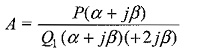Similarly,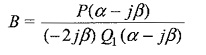In general, B = A* where A* is complex conjugate of A.

If we denote the inverse transform of the complex conjugate terms as f(t)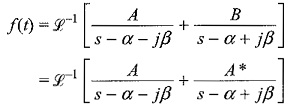where A and A* are conjugate terms.

If we denote A = C + jD, then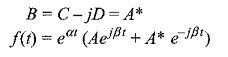Scroll to Top